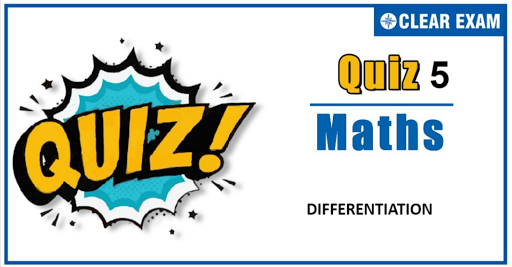## Differentiation Quiz

Important topics for Maths has been designed in such a way that it offers very practical and application-based learning to further make it easier for students to understand every concept or topic by correlating it with the day-to-day experiences.

Q2.Let g(x) be the inverse of an invertible function f(x) which is differentiable for all real x, then g"(f(x)) equals
•  -(f"(x))/(f'(x))^3
•  (f'(x)f''(x)-(f'(x))^3)/(f'(x))
•  (f'(x) f''(x)-(f'(x))^2)/(f'(x))^2
•  None of these
Q3.  d^n/(dx^n )(log⁡x)=
•   (n-1)!/x^n
•  n!/x^n
•  (n-2)!/x^n
•  (-1)^(n-1) (n-1)!/x^n

Q4. If f(x)=|x^2-5x+6|, then f'(x) equals
•  2x-5 for 2
•  5-2x for 2
•  2x-5 for x>2
•  5-2x for x<3
Solution
(b) f(x)=|x^2-5x+6|={x^2-5x+6 if x≥3 or x≤2 -(x^2-5x+6), if 23 or x<2 -(2x-5) if 2

Q5. If u=f(x^3),v=g(x^2),f'(x)=cos⁡x and g'(x)=sin⁡x, then du/dv is
•  3/2 x cos⁡x^3 cos⁡ecx^2
•  2/3 sin⁡x^3 sec⁡x^2
•  tanx
•  None of these
Q6. If y=x-x^2, then the derivative of y^2 with respect to x^2 is
•  1-2x
•  2-4x
• 3x-2x^2
•  1-3x+2x^2
Solution
(d) Let u=y^2 and v=x^2 ∴du/dx=d/dx y^2=(d/dy y^2 )(dy/dx) =2y(1-2x)=2(x-x^2 )(1-2x)=2x(1-x)(1-2x) (1) and dv/dx=2x ...(2) Hence, du/dv=((du/dx))/((dv/dx) )=2x(1-x)(1-2x)/2x (from(1) and (2)) =(1-x)(1-2x)=1-3x+2x^2

•  2/√(x(1-x))
•  Zero
•  -2/√(x(1-x))
•  π
Solution
47 (b) √x=cos⁡θ x∈(0,1/2)⇒√x=cos⁡θ ∈(0,1/√2) ⇒θ∈(π/4,π/2) ⇒2θ∈(π/2,π) ⇒f(x)=2 sin^(-1)⁡ √(1-cos^2⁡θ )+sin^(-1) ⁡(2√(cos^2⁡θ sin^2⁡θ)) =2 sin^(-1)⁡(sin⁡θ)+sin^(-1)⁡(2 sin⁡θ cos⁡θ) =2θ+sin^(-1)⁡(sin⁡2θ) =2θ+π-2θ =π ⇒f'(x)=0

Q8. If f(x) satisfies the relation f((5x-3y)/2)=5f(x)-3f(y)/2 ∀x,yϵ R, and f(0)=3 and f^' (0)=2, then the period of sin(f(x)) is
•
•  π
•
•
Solution
(b) Given f((5x-3y)/2)=(5f(x)-3f(y))/2 ⇒f((5x-3y)/(5-3))=(5f(x)-3f(y))/(5-3), which satisfies section formula for abscissa on L.H.S. and ordinate on R.H.S. Hence,f(x) must be the linear function (as only straight line satisfies such section formula) But f(0)=3⇒b=3,f' (0)=2⇒a=2 Thus, f(x)=2x+3⇒ Period of sin⁡(f(x))=sin⁡(2x+3) is π

Q9.The function f(x)=e^x+x, being differentiable and one to one, has a differentiable inverse f^(-1) (x). The value of d/dx(f^(-1)) at the point f(log⁡2) is
•  1/(In 2)
•  1/3
•  1/4
•  None of these#### Written by: AUTHORNAME

AUTHORDESCRIPTION## Want to know more

Please fill in the details below:

## Latest NEET Articles\$type=three\$c=3\$author=hide\$comment=hide\$rm=hide\$date=hide\$snippet=hide

Name

ltr
item
BEST NEET COACHING CENTER | BEST IIT JEE COACHING INSTITUTE | BEST NEET & IIT JEE COACHING: Differentiation-Quiz-5
Differentiation-Quiz-5